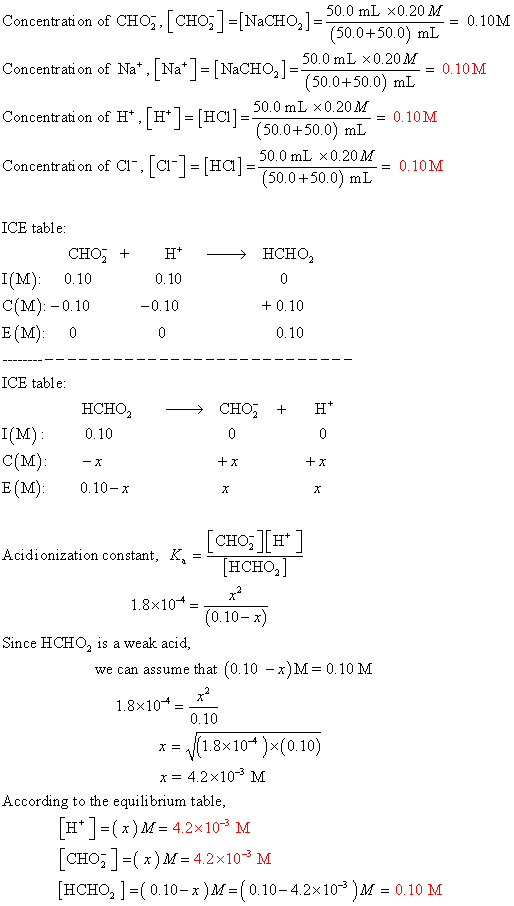Homework Help Question & Answers

# ▼ Part C Calculate the equilibrium concentrations of Na+, Cl ,H+, CHOnd HCHO2 when 50.0 mL of 0.2...▼ Part C Calculate the equilibrium concentrations of Na+, Cl ,H+, CHOnd HCHO2 when 50.0 mL of 0.20 M HCl is mixed with 50.0 mL of 0.20 M NaCHO Express your answers using two significant figures. Enter your answers separated by commas. Request Answer

#### Homework Answers

ReportAnswer #1Add Homework Help Answer
##### Add Answer of: ▼ Part C Calculate the equilibrium concentrations of Na+, Cl ,H+, CHOnd HCHO2 when 50.0 mL of 0.2...
Your Answer: Your Name: What's your source?
Not the answer you're looking for? Ask your own homework help question. Our experts will answer your question WITHIN MINUTES for Free.
More Homework Help Questions Additional questions in this topic.

• #### Calculate equilibrium constant and equilibrium concentrations

Need Online Homework Help?

Get FREE EXPERT Answers
WITHIN MINUTES
Related Questions